Next: Combinatorial Analysis Up: Probability Theory Previous: Combining Probabilities

# Two-State System

The simplest non-trivial system that we can investigate using probability theory is one for which there are only two possible outcomes. (There would obviously be little point in investigating a one-outcome system.) Let us suppose that there are two possible outcomes to an observation made on some system,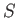. Let us denote these outcomes 1 and 2, and let their probabilities of occurrence be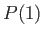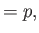(2.9)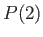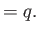(2.10)

It follows immediately from the normalization condition, Equation (2.5), that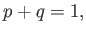(2.11)

so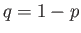. The best known example of a two-state system is a tossed coin. The two outcomes are heads'' and tails,'' each with equal probabilities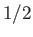. So,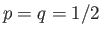for this system.

Suppose that we make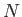statistically independent observations of. Let us determine the probability of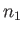occurrences of the outcome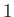, and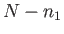occurrences of the outcome 2, with no regard as to the order of these occurrences. Denote this probability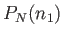. This type of calculation crops up very often in probability theory. For instance, we might want to know the probability of getting nine heads'' and only one tails'' in an experiment where a coin is tossed ten times, or where ten coins are tossed simultaneously.

Consider a simple case in which there are only three observations. Let us try to evaluate the probability of two occurrences of the outcome 1, and one occurrence of the outcome 2. There are three different ways of getting this result. We could get the outcome 1 on the first two observations, and the outcome 2 on the third. Or, we could get the outcome 2 on the first observation, and the outcome 1 on the latter two observations. Or, we could get the outcome 1 on the first and last observations, and the outcome 2 on the middle observation. Writing this symbolically, we have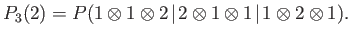(2.12)

Here, the symbolic operator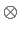stands for and,'' whereas the symbolic operatorstands for or.'' This symbolic representation is helpful because of the two basic rules for combining probabilities that we derived earlier in Equations (2.4) and (2.8):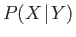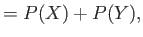(2.13)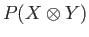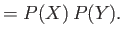(2.14)

The straightforward application of these rules gives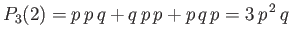(2.15)

for the case under consideration.

The probability of obtainingoccurrences of the outcomeinobservations is given by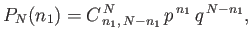(2.16)

where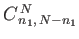is the number of ways of arranging two distinct sets ofandindistinguishable objects. Hopefully, this is, at least, plausible from the previous example. There, the probability of getting two occurrences of the outcome 1, and one occurrence of the outcome 2, was obtained by writing out all of the possible arrangements of twos (the probability of outcome 1) and one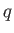(the probability of outcome 2), and then adding them all together.Next: Combinatorial Analysis Up: Probability Theory Previous: Combining Probabilities
Richard Fitzpatrick 2016-01-25